# The Calc Library

The Calc library defines the Limit, Derivative, and Integral objects along with variations on these objects and several supporting objects. It extends commands like Simplify, Expand, etc. to act on the new objects. Integrals and derivatives are calculated using the Execute command.

The Calc library introduces three main objects, the Limit, the Derivative and the Integral. The Derivative and Integral object are created as operators and are used with the On object.

## Derivatives

The Execute command is used to evaluate derivatives. Examples include expressions like:Note that some basic simplification is done on derivatives but they are not necessarily simplified all the way to their simplest form.

The Expand command can be used to evaluate derivatives one step at a time. For example, you get the right-hand side of this expression by applying the Expand command to the left-hand side: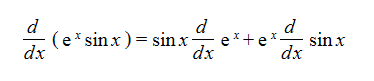which is just an application of the general rule for derivatives of products: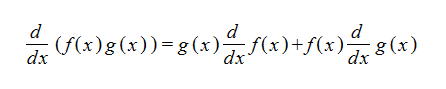## Basic Integrals

The Execute command is also used to evaluate integrals. Examples include expressions like: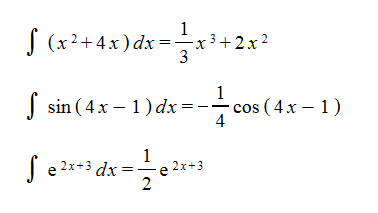Constants and numeric values can be pull out in front of an integral using the Simplify command.

## Integration by Parts

The Execute command on an integral will test to see if the integral has a form that allows evaluation using integration by parts. In addition, you can control how integration by parts is applied to an integral by moving terms into the differential before using the Execute command. For example, if you have the integral: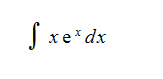you can highlight the e^x term, press Shift + Right arrow to also highlight the dx term and then use the Equivalence command to get:Now you can use the Execute command to get:The Equivalence command can also be used onIt just does a direct application of: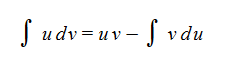## Integration using u-Substitution

Integrals involving u-substitution can be evaluated by highlighting two expressions and applying the Execute command. For example, highlighting these two expressions:and applying the Execute command results in:BETA RELEASE NOTE: The u-substitution functionality is still only partially implemented in the beta version, so there are a relatively limited range of integrals where this approach will work.

## Constants

Constants (for purposes of integration and differentiation) are signified by moving the object to the Constants namespace.

When a derivative or integral is evaluated using the Execute command, it's understood that any term in the Constants namespace should be assumed to be a constant. These terms have a derivative of zero and can be pulled out of integral objects.

In Standard notation, objects in the Constants namespace are rendered in a different color.

BETA RELEASE NOTE:

The range of integrals that can be evaluated in the current beta version is still fairly limited.